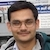# NAEST 2019 Screening Test: Solution to Toy Train QuestionBy

In screening round of National Anveshika Experimental Skills Test ( NAEST 2019 ), a question was asked on a moving toy train. The train moves with a constant speed on a track consisting of (a) straight portion and (b) curved portion. The student needs to assess the acceleration of the train and force on it. The problem statement reads:

Question:

The toy train is moving at a constant speed. Choose the correct option(s)

1. The acceleration of the toy train is zero.
2. The acceleration of the train is non-zero on the straight portion of the track and it is zero on the curved portions.
3. The net force on the train is zero on the straight portion of the track and it is non-zero on the curved portions.
4. The train moves faster on the curved portion of the track and slower on the straight portions.

Solution:

Let us analyse the motion of the train on straight portion of the track. On a straight track, the direction of motion is constant. Thus, speed as well as the direction of motion is constant on the straight track. Hence, the velocity $\vec{v}$ of the train is constant and its acceleration is $\vec{a}=\mathrm{d}\vec{v}/\mathrm{d}t=\vec{0}$. By Newton's second law, $\vec{F}=m\vec{a}$, the net external force on the train (in the horizontal plane) is zero.The velocity of the toy train on the straight path is constant but its direction varies on the curved path.

On the curved portion of the track, the direction of motion varies continuously as the train moves. Although speed is constant, the velocity is not constant because of change in direction of motion. Thus, the acceleration of the train is non-zero. The direction of acceleration is towards the centre of curvature of the track. This acceleration is called centripetal acceleration. By Newton's second law, there is non-zero external force on the train as it moves on the curved path. This force is called centripetal force. This force is provided by the track and it acts at the contact point of the outer wheel.The acceleration of the toy train is zero on the straight path but it is non-zero on the curved path (centripetal acceleration).

The option (D) is also interesting. From the video, it appears that the train moves faster on the curved path as compared to that on the straight path. Since faster or slower depends on the speed of the train, which is given as constant, this option is rejected because it contradicts the problem statement. In many scenario, it is difficult to judge the speed by seeing a moving object. One such scenario is motion of the trees when looking from the window of a moving train.